Quantity: 0

Total: 0,00

0

# Measuring the height of a cathedral

### Related extras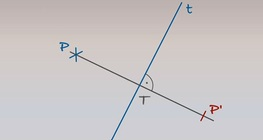#### The reflection of a point across an axis

Axis t and point P are given. Let’s plot the mirror image (point P’) of point P across axis t.#### Perimeter, area, surface area and volume

This animation presents the formulas to calculate the perimeter and area of shapes as...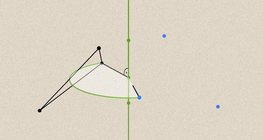#### Geometric transformations – rotation

This animation demonstrates geometric rotation, a type of geometric transformation both...#### Plotting a perpendicular to a line at a given point P on the line

Both line e and point P, which is situated on it, are given. Let’s plot line g to include point...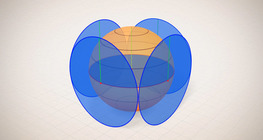#### Surface area of spheres (demonstration)

The surface of a sphere consists of the set of points which are all at the same distance...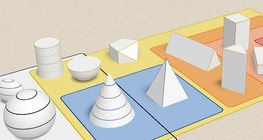#### Grouping of solids 3

This animation demonstrates various groups of solids through examples.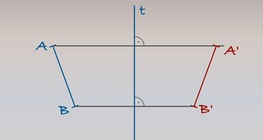#### Reflecting a line segment across an axis

Axis t and line segment AB are given on a plane. Let’s plot the mirror image of line segment AB...#### Grouping of cuboids

This animation demonstrates various types of cuboids through everyday objects.

Added to your cart.